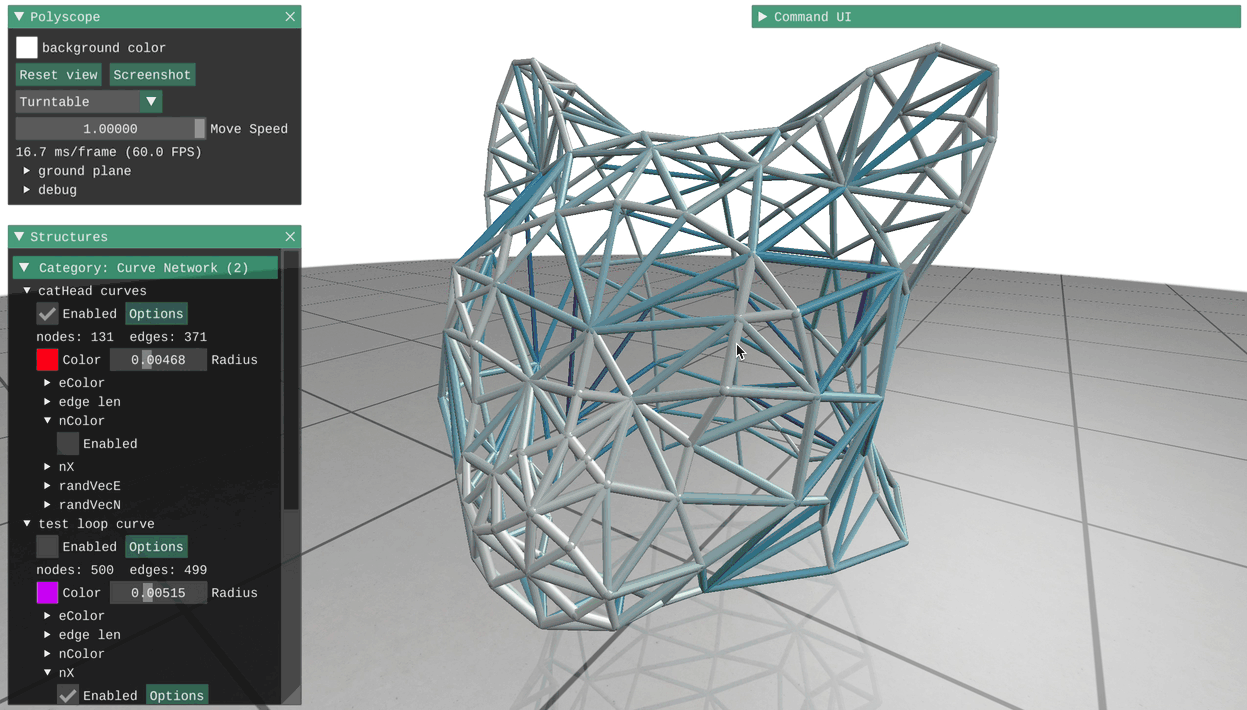# Curve Networks

Curve networks are collections of nodes sitting in space, connected by edges. In addition to displaying the nodes and edges of the network itself, Polyscope can show any number of scalar, vector, or color quantities associated with the nodes or edges of the network.

Try clicking on a node or edge to see the data associated with that point!### Registering a curve network

Curve network structures can be registered with Polyscope by passing the node position and edge indices. Polyscope also makes it easy to automatically construct lines and loops by just passing edges='line' or edges='loop'.

Example: a network of random curves

import numpy as np
import polyscope as ps
ps.init()

# generate some random nodes and edges between them
nodes = np.random.rand(100, 3)
edges = np.random.randint(0, 100, size=(250,2))

# visualize!
ps_net = ps.register_curve_network("my network", nodes, edges)
ps.show()


register_curve_network(name, nodes, edges, enabled=None, radius=None, color=None, material=None)

Add a new curve network structure to Polyscope.

• name string, a name for the structure
• nodes, an Nx3 numpy float array of node locations (or Nx2 for 2D)
• edges, an Ex2 numpy integer array of edge connections, as 0-based indices in to the nodes array, OR the string line/loop, to generate node connectivity as a line or loop, respectively.

• enabled boolean, is the structure enabled initially
• radius float, a size for the nodes and edges relative to the scene length scale (use set_radius(val, relative=False) for absolute units)
• color float 3-tuple, default color values for the network as rgb in [0,1]
• material string, name of material to use for network

if not specified, these optional parameters will assume a reasonable default value, or a persistant value if previously set.

2D node positions are also supported, see 2D data.

### Updating a curve network

The locations of the nodes in a curve network can be updated with the member function update_node_positions(newPositions). All quantities will be preserved. Changing the connectivity or number of nodes/edges is not supported, you will need to register a new curve network (perhaps with the same name to overwrite).

CurveNetwork.update_node_positions(newPos)

Update the node positions in a curve network structure. newPos must be valid input as to initially construct the nodes of the network, with the same number of nodes as the network curently has.

### Options

Options control the appearance of the network . Note that these options can also be passed as keyword arguments to the initial register_curve_network(), as noted above.

Parameter Meaning Getter Setter Persistent?
enabled is the structure enabled? is_enabled() set_enabled(newVal=True) yes
radius size of rendered nodes/edges get_radius() set_radius(newVal, relative=True) yes
color default color for the network get_color() set_color(newVal) yes
material material for structure get_material() set_material(newVal) yes

Example: set options which affect the appearance of the curve network

network = polyscope.register_curve_network("my net", nodes, edges)

network.set_enabled(False) # disable
network.set_enabled() # default is true

network.set_radius(0.02) # radius is relative to a scene length scale by default